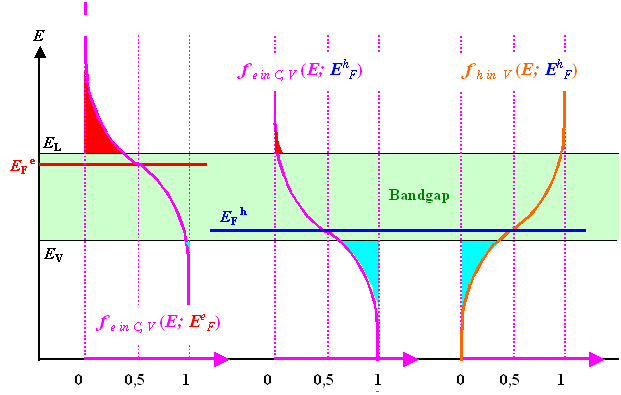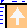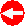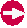### 2.3.2 Quasi Fermi EnergiesSo far we implicitly defined (thermal) equilibrium as a total equilibrium involving three components if you think about it:1. Equilibrium of the electrons in the conduction band. This means their density was given (within the usual approximations) by
ne  = Neffe  ·  exp – ECEF
kT2. Equilibrium of the holes in the valence band. This means their density was given (within the usual approximations) by
nh   = Neffh  ·  exp – EFEV
kT3. Equilibrium between the electrons and holes, i.e. between the bands. This means that the Fermi energy is the same for both bands (and positioned somewhere in the band gap).

Definition of the Quasi Fermi EnergyIf an equilibrium is disturbed, it takes a certain time before it is restored again; this is described by the kinetics of the processes taking place. In a strict sense of speaking, the Fermi energy is not defined without equilibrium, but only after it has been restored. This restoring process occurs in the bands and between the bands:In the bands, local equilibrium (in k-space) between the carriers will be obtained after there was time for some collisions, i.e. after some multiples of the scattering time. This process is known as thermalization and occurs typically in picoseconds.Between the bands, equilibrium will be restored by generation and recombination events and this takes a few multiples of the carrier life time, i.e. at least nanoseconds if not milliseconds.This means that we can have a partial (or local) equilibrium in the bands long before we have equilibrium between the bands. This local equilibrium implies that:Non-equilibrium means something changes in time. Changes in the properties of the particle ensemble considered (i.e. electrons and holes) in local equilibrium are only due to "traffic" between the bands while the properties of the particles in the band do not change anymore. The term "local" of course, does not refer to a coordinate, but to a band.The carrier densities therefore do not have their total or global equilibrium value as given, e.g., by the mass action law, but their local equilibrium density can still be given in terms of the equilibrium distribution by
n e  =  N effe  · exp – E CEF e
kT

nh  =  N effh  · exp – EFhEV
kTwith the only difference that the Fermi energy now is different for the electrons and holes. Instead of one Fermi energy EF for the whole system, we now have two Quasi Fermi energies, EFe and EFh.For the product of the carrier densities we now obtain a somewhat modified mass-action law
ne · nh   =  Neffe  · Neffh  · exp – (ECE V) + (EFh EFe)
kT
=   ni2 · exp – EFhE F e
kTFor this we used the by now basic relation
Neffe  · Neffh  · exp – ECEV
kT
=  ni 2The name "Quasi Fermi energy" is maybe not so good, there is nothing "quasi " about it. Still, that's the name we and everybody else will use. Sometimes it is also called "Imref" (Fermi backwards), but that doesn't help much either.Rewriting the equations from above gives a kind of definition for the Quasi Fermi energies:
EF e   =  EC  –  kT · ln Neffe
n e

EFh  =  EV  +  kT · ln N effh
nhQuasi Fermi energies are extremely helpful for the common situation where we do have non-equilibrium, but only between the bands – and that covers most of semiconductor devices under conditions of current flow (due to an applied voltage) or under illumination. We will make frequent use of Quasi Fermi energies!

Carrier Densities and Quasi Fermi EnergiesIf we calculate carrier densities in non-equilibrium with the Quasi Fermi energies, we have to be careful to use the right Quasi Fermi energy in the Fermi-Dirac formula or in the Boltzmann approximation.After all, we now have two (Quasi) Fermi energies, one "regulating" the density of electrons in the conduction band, and the other one doing the same for the holes in the valence band. That was already implied above, here we want to make this topic a bit clearer; we also introduce a new distribution function as a kind of short-hand.You really must now write f(E, EFe , T) or f(E, EFh, T) instead of simply f(E, EF, T) or f(E) because, due the different arguments, the meaning of these two expressions is now different. This is illustrated below with the two curves on the left and should be obvious.In the pictures we even have some redundancy by writing fe in C(E, EFe, T) and so on. This is not necessary, but helps in the beginning to avoid mix-up.The density of electrons or holes in the conduction or valence band, respectively, would now be
ne  =  Neffe · [fe in C(E, EF e, T)]   »   Neffe  · exp – ECEF e
kT

nh  =  Neffh · [1 – fe in V(E, EFh, T)]   »   Neffh  · exp – EFhEV
kTThe red or blue triangles above symbolize the density of electrons in the conduction band or holes in the valence band, respectively, as before.The right-hand side is identical (of course) to what we had above and shows a kind of symmetry not contained in the formulation with the Fermi distribution, where we have f(E, EF h, T) and 1 – f(E, EFh, T).This can be remedied easily by simply setting 1 – f(E, E Fh, T) =: fh in V(E, EFh, T) with f h in V being the probability of finding holes on the available states in the valence band.This is the curve shown on the right-hand side in the picture above.If we use that definition, we obtain more symmetry at the cost of more heavily indexed functions. It's a matter of taste.However, later we will encounter situations where proper bookkeeping of electrons and holes is complicated and essential. Then it might be easier to keep the situation symmetric, to use fh in V for the holes in the valence band, and to express all carrier densities in the valence band with fh in V, while in the conduction band we use fe in C.© H. Föll (Semiconductors - Script)